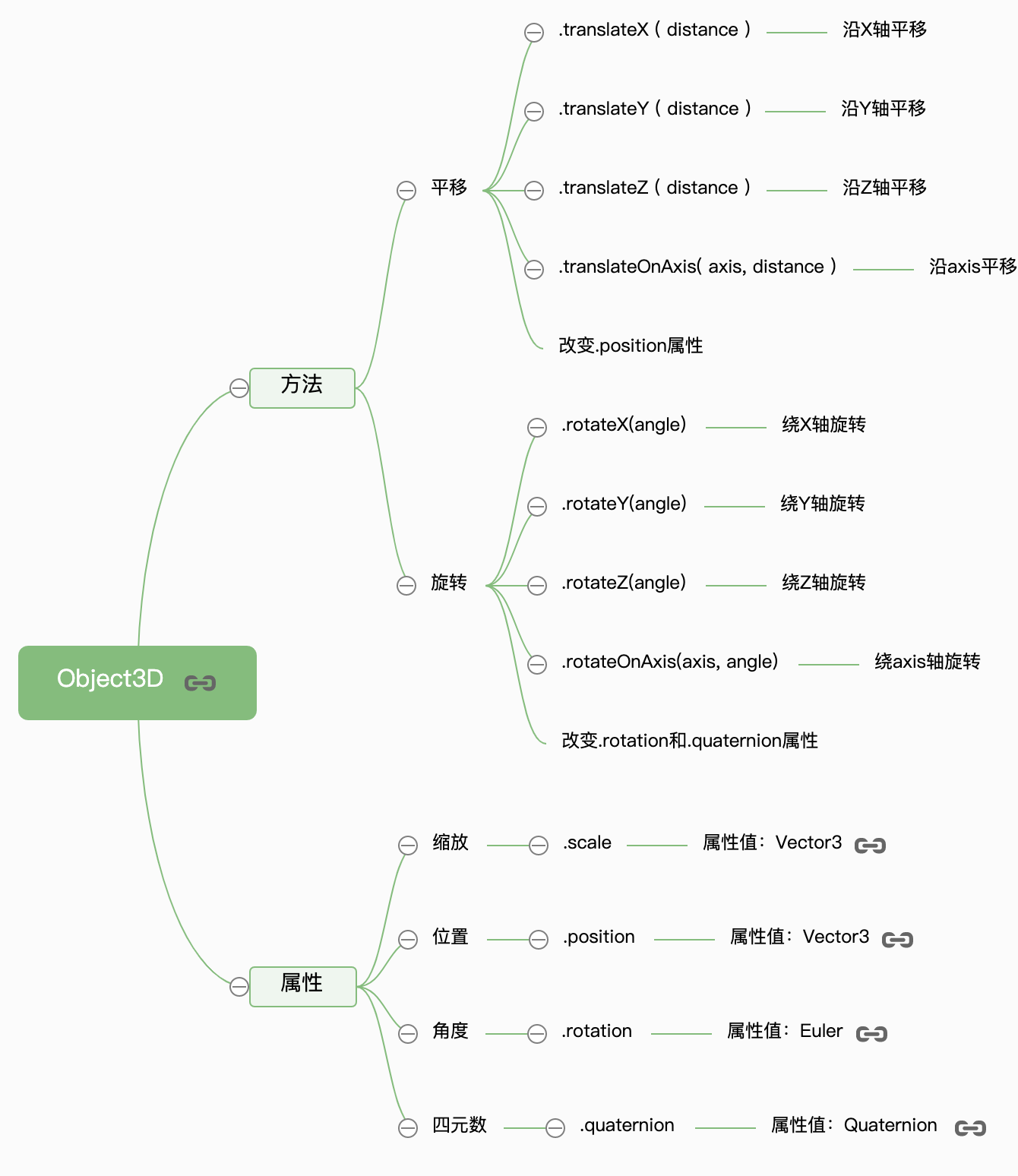### 缩放

`mesh.scale.set(0.5, 1.5, 2)`

x轴方向放大2倍

`mesh.scale.x = 2.0;`

### 设置网格模型y坐标

``mesh.position.y = 80;``

### 设置模型xyz坐标

``mesh.position.set(80,2,10);``

### 平移

``````// 等价于mesh.position = mesh.position + 100;
mesh.translateX(100);//沿着x轴正方向平移距离100``````

``mesh.translateZ(-50);``

``````//向量Vector3对象表示方向
var axis = new THREE.Vector3(1, 1, 1);
axis.normalize(); //向量归一化
//沿着axis轴表示方向平移100
mesh.translateOnAxis(axis, 100);``````

### 旋转

``mesh.rotateX(Math.PI/4);//绕x轴旋转π/4``

``````var axis = new THREE.Vector3(0,1,0);//向量axis
mesh.rotateOnAxis(axis,Math.PI/8);//绕axis轴旋转π/8``````

``````// 绕着Y轴旋转90度
mesh.rotateY(Math.PI / 2);
//控制台查看：旋转方法，改变了rotation属性
console.log(mesh.rotation);``````